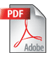Intermediate Math League of Eastern Massachusetts > Meet Information >
IMLEM Round Topics 2019-20

## Meet #1 -- October 17, 2019 -- No CalculatorsRound 1. Mystery Round 2. Geometry -- Angle measures in plane figures, including supplements and complements Round 3. Number Theory -- Divisibility rules; factors; primes and composites Round 4. Arithmetic -- Order of operations; mean, median, mode; rounding; statistics Round 5. Algebra -- Simplifying and evaluating expressions; solving equations with one unknown, including identities Team Round. All of the above (five questions) plus one additional question

## Meet #2 -- November 21, 2019 -- No CalculatorsRound 1. Mystery Round 2. Geometry -- Area and perimeter of polygons Round 3. Number Theory -- GCF, LCM; prime factorization Round 4. Arithmetic -- Fractions; terminating and repeating decimals; percents Round 5. Algebra -- Word problems with one unknown; working with formulas; reasoning in number sentences Team Round. All of the above (five questions) plus one additional question

## Meet #3 -- January 16, 2020 -- No CalculatorsRound 1. Mystery Round 2. Geometry -- Properties of polygons; Pythagorean Theorem Round 3. Number Theory -- Bases; scientific notation Round 4. Arithmetic -- Integral powers (positive, negative, and zero); roots up to the sixth Round 5. Algebra -- Absolute value; inequalities in one variable, including interpreting line graphs Team Round. All of the above (five questions) plus one additional question

## Meet #4 -- February 27, 2020 -- Calculator MeetRound 1. Mystery Round 2. Geometry -- Properties of circles Round 3. Number Theory -- Modular arithmetics; series and sequences Round 4. Arithmetic -- Percent applications; percent change; compound interest Round 5. Algebra -- Word problems (linear, including direct proportions or systems) Team Round. All of the above (five questions) plus one additional question

## Meet #5 -- April 2, 2020 -- Calculator Meet

 Round 1. Mystery Round 2. Geometry -- Solid geometry (volume and surface area) Round 3. Number Theory -- Set theory; Venn diagrams Round 4. Arithmetic -- Combinatorics and probability Round 5. Algebra -- Solving quadratics with rational solutions, including word problems Team Round. All of the above (five questions) plus one additional question
(The April meet was cancelled because of COVID-19 school closures.)